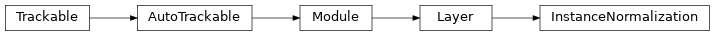InstanceNormalization¶

Inheritance Diagramclass ashpy.layers.instance_normalization.InstanceNormalization(eps=1e-06, beta_initializer='zeros', gamma_initializer='ones')[source]

Bases: tensorflow.python.keras.engine.base_layer.Layer

Instance Normalization Layer (used by Pix2Pix  and Pix2PixHD  ).

Basically it’s a normalization done at instance level. The implementation follows the basic implementation of the Batch Normalization Layer.

• Direct Usage:

x = tf.ones((1, 10, 10, 64))

# instantiate attention layer as model.
normalization = InstanceNormalization()

# evaluate passing x.
output = normalization(x)

# the output shape is.
# the same as the input shape.
print(output.shape)

• Inside a Model:

def MyModel():
inputs = tf.keras.layers.Input(shape=[None, None, 64])
normalization = InstanceNormalization()
return tf.keras.Model(inputs=inputs, outputs=normalization(inputs))

x = tf.ones((1, 10, 10, 64))
model = MyModel()
output = model(x)

print(output.shape)

(1, 10, 10, 64)

  Image-to-Image Translation with Conditional Adversarial Networks https://arxiv.org/abs/1611.07004
  High-Resolution Image Synthesis and Semantic Manipulation with Conditional GANs https://arxiv.org/abs/1711.11585

Methods

 __init__([eps, beta_initializer, …]) Initialize the layer. build(input_shape) Assemble the layer. call(inputs[, training]) Perform the computation.

Attributes

 activity_regularizer Optional regularizer function for the output of this layer. dtype dynamic inbound_nodes Deprecated, do NOT use! Only for compatibility with external Keras. input Retrieves the input tensor(s) of a layer. input_mask Retrieves the input mask tensor(s) of a layer. input_shape Retrieves the input shape(s) of a layer. input_spec losses Losses which are associated with this Layer. metrics name Returns the name of this module as passed or determined in the ctor. name_scope Returns a tf.name_scope instance for this class. non_trainable_variables non_trainable_weights outbound_nodes Deprecated, do NOT use! Only for compatibility with external Keras. output Retrieves the output tensor(s) of a layer. output_mask Retrieves the output mask tensor(s) of a layer. output_shape Retrieves the output shape(s) of a layer. submodules Sequence of all sub-modules. trainable trainable_variables Sequence of variables owned by this module and it’s submodules. trainable_weights updates variables Returns the list of all layer variables/weights. weights Returns the list of all layer variables/weights.
__init__(eps=1e-06, beta_initializer='zeros', gamma_initializer='ones')[source]

Initialize the layer.

Parameters: eps (float) – Variance_epsilon used by batch_norm layer. beta_initializer (str) – Initializer for the beta variable. gamma_initializer (str) – Initializer for the gamma variable. None
build(input_shape)[source]

Assemble the layer.

Parameters: input_shape (tuple of (int)) – Specifies the shape of the input accepted by the layer. None
call(inputs, training=False)[source]

Perform the computation.

Parameters: inputs (tf.Tensor) – Inputs for the computation. training (bool) – Controls for training or evaluation mode. Tensor tf.Tensor – Output Tensor.